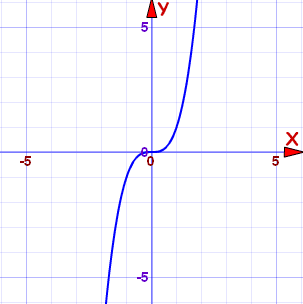# Cube Function

This is the Cube Function:

f(x) = x3

This is its graph:f(x) = x3

It flattens out at (0,0)

It has origin symmetry.

And it is an odd function.

Its Domain is the Real Numbers:Its Range is also the Real Numbers:Plot the graph here